Courses

# MCQ(Previous Year Questions) - Structure Of Atom (Level 2)

## 18 Questions MCQ Test Chemistry for JEE Advanced | MCQ(Previous Year Questions) - Structure Of Atom (Level 2)

Description
This mock test of MCQ(Previous Year Questions) - Structure Of Atom (Level 2) for JEE helps you for every JEE entrance exam. This contains 18 Multiple Choice Questions for JEE MCQ(Previous Year Questions) - Structure Of Atom (Level 2) (mcq) to study with solutions a complete question bank. The solved questions answers in this MCQ(Previous Year Questions) - Structure Of Atom (Level 2) quiz give you a good mix of easy questions and tough questions. JEE students definitely take this MCQ(Previous Year Questions) - Structure Of Atom (Level 2) exercise for a better result in the exam. You can find other MCQ(Previous Year Questions) - Structure Of Atom (Level 2) extra questions, long questions & short questions for JEE on EduRev as well by searching above.
QUESTION: 1

### The energy of an electron in the first Bohr orbit of H atom is -13.6 eV. The possible energy value(s) of the excited state(s) for electrons in Bohr orbits of hydrogen is/are :                                          [JEE 1998]

Solution: Obviously during the excited state the electron will jump to higher shell.i.e 2,3..Since the energy is quantised the exact energy required to jump to 2 shell is -3.4j and no other given value is quantised or of exact value to jump to any other shell.
*Multiple options can be correct
QUESTION: 2

### Which of the following statement(s) is (are) correct ?                                         [JEE 1998]

Solution:
*Multiple options can be correct
QUESTION: 3

### Assertion: Zn2+ is diamagnetic.                                             [JEE 1998] Reason: The electrons are lost from 4s orbital to form Zn2+.

Solution:
*Multiple options can be correct
QUESTION: 4

The electrons, identified by quantum numbers n and l

(i) n = 4, l = 1,

(ii) n = 4, l = 0

(iii) n = 3, l = 2 and

(iv) n = 3, l = 1

can be placed in order of increasing energy, from the lowest highest, as                   [JEE 1999]

Solution:
QUESTION: 5

Electronic configuration of an element is 1s22s22p63s 23p63d54s1. This represents its                            [JEE 2000]

Solution: It represent chromium in it's ground state
QUESTION: 6

The wavelength associated with a golf ball weighing 200 g and moving at a speed of 5 m/h is of the order

[JEE 2001]

Solution: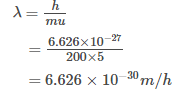Hence (c) is the correct answer.
QUESTION: 7

The number of nodal planes in a px orbital is :                                           [JEE 2000]

Solution:
No of nodal planes in an orbital = l [azimuthal quantum number]
For p orbital l = 1
Hence Nodal plane in p-orbital =1
Hence (a) is the correct answer.
QUESTION: 8

The quantum numbers +1/2 and -1/2 for the electron spin represent :                                     [JEE 2001]

Solution:
QUESTION: 9

Rutherfords experiment, which established the nuclear model of atom, used a beam of :                                  [JEE 2002]

Solution: He uses alpha rays because of its high ionising power and low penetrating power.Alpha ray is basically a beam of fast moving of helium nuclei.
QUESTION: 10

The radius of which of the following orbit is same as that of the first Bohr's orbit of hydrogen atom?   [JEE 2004]

Solution:
QUESTION: 11

The number of radial nodes of 3s and 2p orbitals are respectively.                                               [JEE 2004]

Solution: Total radial node =n-l-1 3-0-1=2 2-1-1=0
*Multiple options can be correct
QUESTION: 12

Ground state electronic configuration of nitrogen atom can be represented by           [JEE 1999]

Solution:
QUESTION: 13

Assuming that Hund's rule is violated, the bond order and magnetic nature of the diatomic molecular B2 is                                   [JEE 2010]

Solution:
QUESTION: 14

The hydrogen-like species Li2+ is in a spherically symmetric state S1 with one radial node. Upon absorbing light the ion undergoes transition to a state S2. The state S2 has one radial node and its energy is equal to the ground state energy of the hydrogen atom.  [JEE 2010]

Q. The state S1 is

Solution:
QUESTION: 15

The hydrogen-like species Li2+ is in a spherically symmetric state S1 with one radial node. Upon absorbing light the ion undergoes transition to a state S2. The state S2 has one radial node and its energy is equal to the ground state energy of the hydrogen atom.                  [JEE 2010]

Q. Energy of the state S1 in units of the hydrogen atom ground state energy is

Solution:
QUESTION: 16

The hydrogen-like species Li2+ is in a spherically symmetric state S1 with one radial node. Upon absorbing light the ion undergoes transition to a state S2. The state S2 has one radial node and its energy is equal to the ground state energy of the hydrogen atom.                            [JEE 2010]

Q. The orbital angular momentum quantum number of the state S2 is

Solution:
QUESTION: 17

Given in hydrogenic atom rn, Vn, E, Kn stand for radius, potential energy, total energy and kinetic energy in nth orbit. Find the value of U, v, x, y.                           [JEE 2006]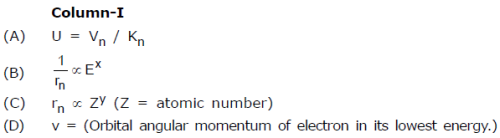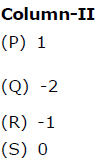Solution: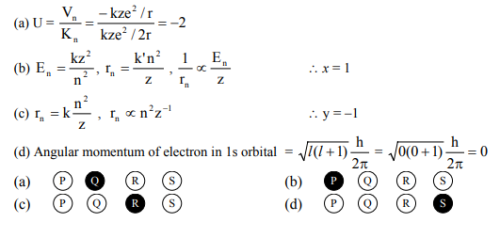QUESTION: 18

Match the entries is Column-I with the correctly related quantum number(s) in Column-II. Indicate your answer by darkening the appropriate bubbles of the 4 × 4 matrix given in the ORS.             [JEE 2008]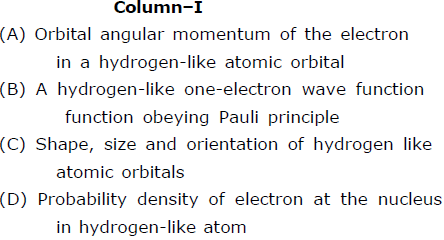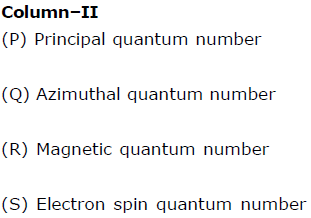Solution: